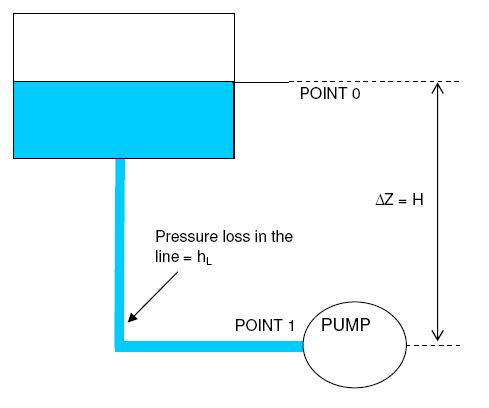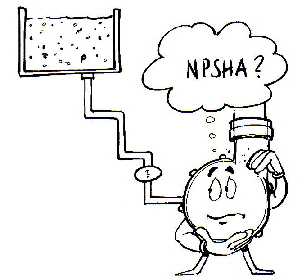## NPSHa CalculatorImperial Units
Metric Units
Altitude above sea level:
ft
meters
Atmospheric pressure at given altitude:
ft w.g.
kPa
Leaving Water Temperature (LWT):
°F
°C
Water vapor pressure at LWT°:
ft w.g.
kPa
Friction losses in suction pipe:
ft w.g.
mm w.g.
Height "H" (see image):
ft
mm
NPSHa (available at pump's suction)
inches
mm w.g.#### Notes:

1. Fill out the green spaces only.
2. "Height" is the elevation difference between the pump inlet and the water surface in the tower
3. Although "H" is not equal to the foundation height, we suggest to use it as such, in order to have some safety factor built into the calculation
4. Calculated NPSHa must be higher than the pump's NPSHa at duty point
5. If the calculated NPSHa is insufficient, increase the height until the NPSHr is satisfied (NPSHa > NPSHr)
6. Do not input negative values for "H" (flooded suction)